# High School Math : How to find out if lines are parallel

## Example Questions

### Example Question #1 : How To Find Out If Lines Are Parallel

Which of these lines is parallel to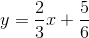?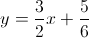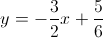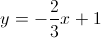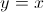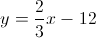Explanation:

Lines are parallel if they have the same slope. In standardform,is the slope.

For our given equation, the slope is. Only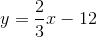has the same slope.

### Example Question #2 : How To Find Out If Lines Are Parallel

Which of the following lines is parallel to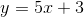?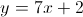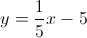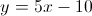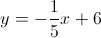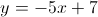Explanation:

Two lines that are parallel have the same slope. The slope ofis, so we want another line with a slope of. The only other line with a slope ofis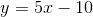### Example Question #3 : How To Find Out If Lines Are Parallel

Which of these lines is parallel to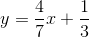?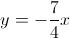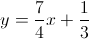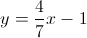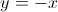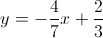Explanation:

Lines are parallel if they have the same slope. In standardform,is the slope.

For our given equation, the slope is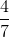. Only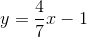has the same slope.

### Example Question #4 : Parallel Lines

Which of the following lines will be parallel to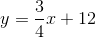?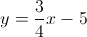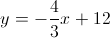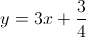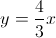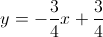Explanation:

Two lines are parallel if they have the same slope. When a line is in standardform, theis the slope.

For the given line, the slope will be. Only one other line has a slope of: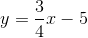### Example Question #5 : Parallel Lines

Are the following lines parallel?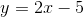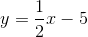It cannot be determined from the information given

Yes

No

No

Explanation:

By definition, two lines are parallel if they have the same slope. Notice that since we are given the lines in theformat, and our slope is given by, it is clear that the slopes are not the same in this case, and thus the lines are not parallel.

### Example Question #6 : Parallel Lines

Which of the following lines is parallel to the line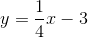?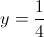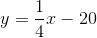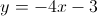Explanation:

Parallel lines have the same slope. In slope-intercept form,,is the slope.Here the slope is; thus, any line that is parallel to the line in question will also have a slope of.

Only one answer choice satisfies this requirement: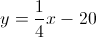Note: the answer choice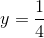is incorrect. If put intoform, the equation becomes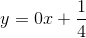.  Therefore the slope is actually, not.